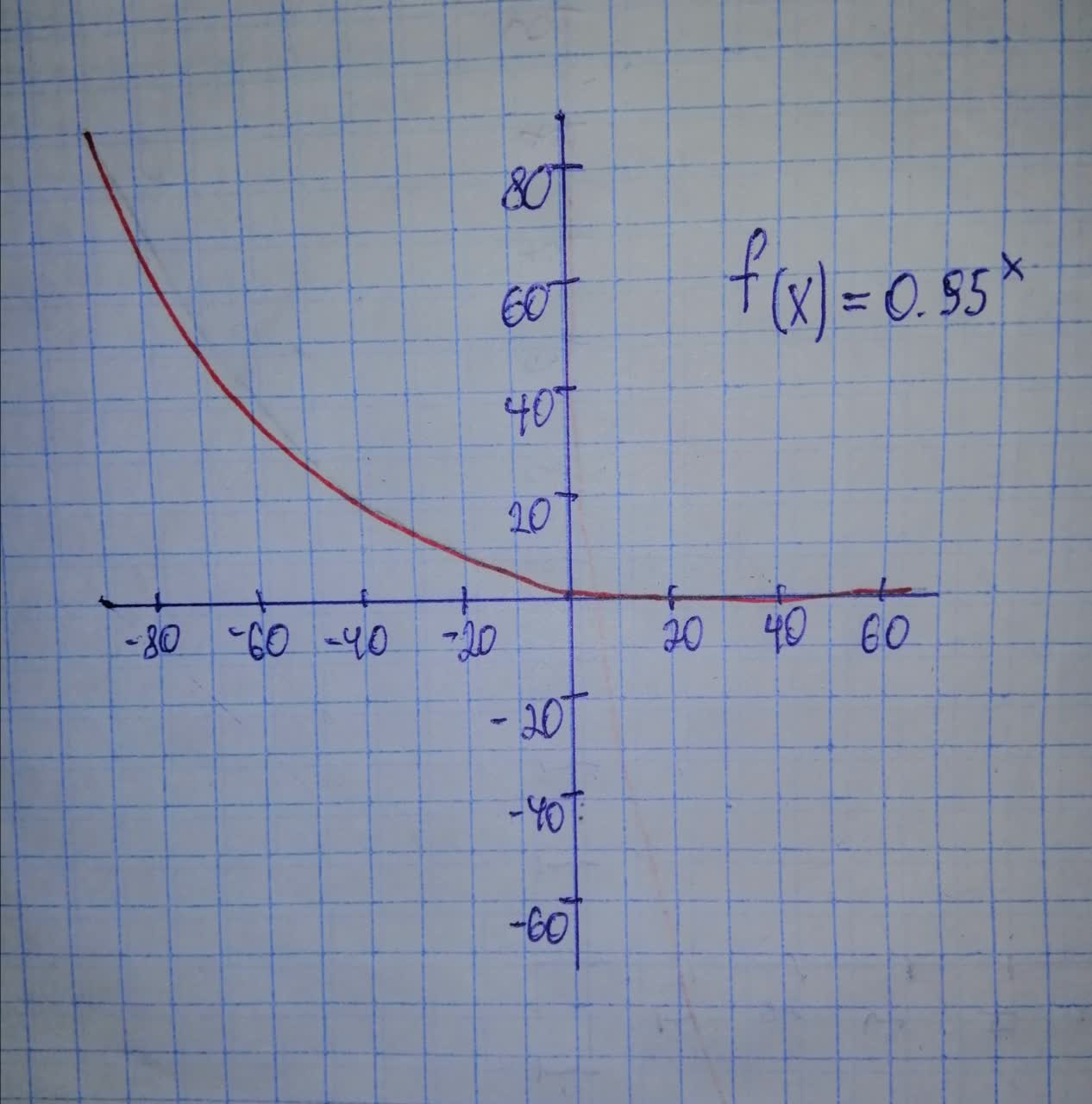# Tell whether the function represents exponential growth or exponential decay. Then graph the function. y=(0.95)^{x}defazajx 2020-12-28 Answered
Tell whether the function represents exponential growth or exponential decay. Then graph the function.
$y={\left(0.95\right)}^{x}$
You can still ask an expert for help

## Want to know more about Exponential growth and decay?

• Questions are typically answered in as fast as 30 minutes

Solve your problem for the price of one coffee

• Math expert for every subject
• Pay only if we can solve itAGRFTr
Step 1
Given function
$y={\left(0.95\right)}^{x}$
Step 2
Graph